# HBSE 7th Class Maths Solutions Chapter 11 Perimeter and Area InText Questions

Haryana State Board HBSE 7th Class Maths Solutions Chapter 11 Perimeter and Area InText Questions and Answers.

## Haryana Board 7th Class Maths Solutions Chapter 11 Perimeter and Area InText Questions

Try These (Page 205)

Question 1.
What would you need to find area or perimeter, to answer the following?
1. how much space does a black-board occupy?
2. What is the length of wire require to fence a rectangular flower bed?
3. What distance would you cover by taking two rounds of a triangular park?
4. How much plastic sheet do you need to cover a rectangular swimming pool?
Solution:
1. Perimeter of rectangular black board = 2 x (l + b)
2. Length of the wire = Perimeter of rectangular flower bed = 2 x (l + b)
3. Perimeter of rectangular park = 2(l + b).
4. Perimeter ofrectangular swimming pool = 2(l+b).

Try These (Page 206)

Question 1.
Experiment with several such shapes and cut-outs. You might find it useful to draw these shapes on squared sheets and compute their area and perimeter.
You have seen that increase in perimeter does not mean that area will also increase.
Question 2. Give two examples where the area increases as the perimeter increases.
Question 3. Give two examples where the area does not increase when perimeter increases.
Solution:
1. Area of rectangle = l × b
= 3 cm × 2 cm
= 6 cm2.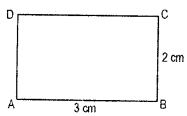Perimeter of rectangle = 2 (l + b)
= 2 (3 + 2) = 2 × 5 = 10 cm. 2.

2. (a) Area of rectangle = l × b
= 4 cm × 3 cm = 12 cm2.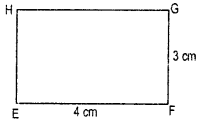Perimeter of rectangle = 2(l + b)
= 2 (4 cm + 3 cm)
= 2 × 7 cm = 14 cm.

(b) Area of square (Fig.) = Side × side = 2 cm × 2 cm = 4 cm2.
Perimeter of square = 4 × side = 4 × 2 cm = 8 cm.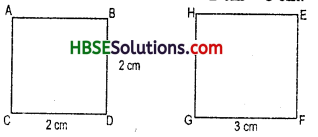3. Area of square = 3 cm × 3 cm = 9 cm2
Perimeter of square = 4 × 3 cm = 12 cm.
Hence area decreases as the perimeter increases.Try These (Page 210)

Question 1.
Each of the following rectangles of the length 6 cm and the breadth 4 cm is composed of congurent polygons. Find the area of each polygon.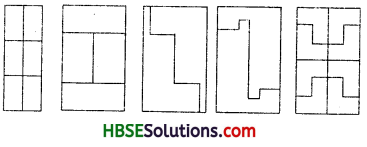Solution:
Since, the two parts are congruent to each other.
So, the area of any part is equal to the area of the other part.
Therefore the area of congurent polygons 1
= $$\frac{1}{2}$$ (The area of rectangle)
= $$\frac{1}{2}$$ (6 cm × 4 cm) = $$\frac{1}{2}$$ × 24 cm2 = 12 cm2

Try These (Page 212)

Question 1.
Find the area of following parallelograms :
(i)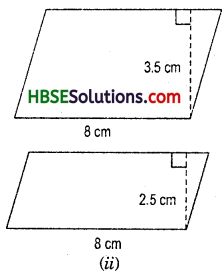(ii) In a parallelogram ABCD, AB = 7.2 cm and the prependicular from con AB is 4.5 cm.
Solution:
Area of parallelogram = base × height
(i) Here, base = 8 cm,
height = 3.5 cm
∴ Area = 8 × 3.5 = 28.0 cm2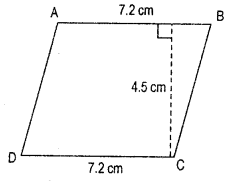(ii) base = 8 cm,
height = 2.5 cm
Area = 8 × 2.5 = 20.0 cm2

(iii) Here base = 7.2 cm
height = 4.5 cm
(ii) Area of parallelogram = base × height
= 7.5 × 2.5 = 32.40cm2Try These (Page 213)

Question 1.
Try the above activity with dirrerent types of triangles.
Solution:
Area of parallelogram = base × height
or, Area of parallelogram
= [ $$\frac{1}{2}$$ × b × h + $$\frac{1}{2}$$ × b × h ]
i.e. In a parallelogram,
Area of each triangle = $$\frac{1}{2}$$ (b × h)

Question 2.
Take different paralelograms. Divide each of the parallelograms into two triangles by cutting along any of its diagonals. Are the triangles congruent?
Solution:
In a parallelogram have two triangle, ΔABD and ΔBDC.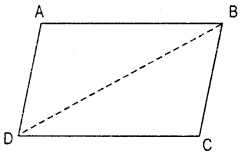In a parallellogram,
AB = DC andAD = BC
and AB || DC and AD || BC.
Hence, AB = DC, B = BD (common)
∴ ∆ABD ≅ ∆BDC (SSS congruency)
So, by CPCT all angles are equal.

Try These (Page 219)

Question 1.
(a) Which square has the larger perimeter ?
(b) Which is larger, perimeter of smaller square or the circumference of the circle?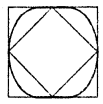Solution:
(a) Perimeter of square = 4 × side According to fig. outer square is larger perimeter than inner square.
(b) Perimeter or circumference of circle
= 2πr [π = 22/7]
and perimeter of square = 4 × side
If side of square = diameter of circle = 14 cm
hence radius of circle = $$\frac{14}{2}$$ = 7 cm
Now, Perimeter of square = 4 × 7 = 28 cm
and circumference of circle = 2 × $$\frac{22}{7}$$ × 7
= 44 cm.
Hence, perimeter of square is smaller than circumference of circle.Try These (Page 222)

Question 1.
Draw circles of different radii on a graph paper. Find the area by counting the number of squares. Also find the area by using the formula. Compare the two amswer.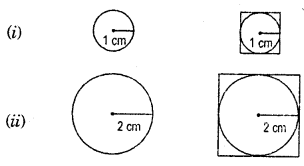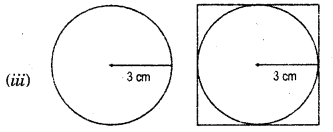Solution:
(i) radius of circle = 1 cm
d = 2cm
hence, side of square = 2 cm
Area of square = (side)2
= (2)2 = 4 cm2

(ii) r = 2 cm, ∴ d = 4 cm
Area of Square = (4)2 = 16cm2

(iii) r = 3 cm, ∴ d = 6 cm
Area of Square = (6)2 = 36 cm2
If the area by counting the number of square and compare the answer, we get.
Area circle = Area of Square

Try These (Page 225)

Question 1.
Convert the following:
(i) 50 cm2 in mm2
(ii) 50 ha in m2
(Hi) 110 m2 in cm2
(iv) 1,000 cm2 in m2
Solution:
(i) ∵ 50 cm2 = 50 × 100 mm2
= 5000 mm2

(ii) ∵ 1 hectare = 10,000 m2
∴ 2 hectare = 2 × 10,000 m2
= 20,000 m2

(iii) ∵ 1 m2 = 10,000 cm2
∴ 10 m2 = 10 × 10,000 cm2
= 1,00,000 cm2(iv) ∵ 10000 cm2 = 1 m2
∴ 12 = $$\frac{1}{10000}$$m2
∴ 1000 cm2 = $$\frac{1}{10000}$$ m2 × 1,000
= $$\frac{1}{10}$$cm2 = 0.1 m2